# Draw a labelled ray diagram to show the formation of an image by a compound microscope. Write the expression for its magnifying power. How does the resolving power of a compound microscope change, when refractive index of the medium between the object and the objective lens increases; and wavelength of the radiation used is increased? An electric dipole is held in a uniform electric field. Using suitable diagram, show that it does not undergo any translatory motion and derive an expression for the torque acting on it and specify its direction. Draw a graph to show the variation of the angle of deviation 'D' with that of the angle of incidence 'i' for a monochromatic ray of light passing through a glass prism of refracting angle 'A'. Hence deduce the relation.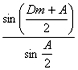Two long parallel straight wires X and Y separated by a distance of 5 cm in air carry currents of 10 A and 5 A respectively in opposite directions. Calculate the magnitude and direction of the force on a 20 cm length of the wire Y.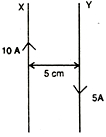A circcircular coil of100 turns, radius 10 cm carries a current of 5 A. it is suspended vertically in a uniform horizontal magnetic field of 0.5 T, the field lines making an angle of 600 with the of the plane of the coil. Calculate of the torque must be applied on it to prevent it from turning. Two point charges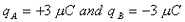are located 20 cm apart in vacuum, Find the electric field at the mid point of the line AB joining the two charges, If a negative test charge of magnitude 1.5 x10-9c is placed at the centre, find the force experienced by the test charge. In a given circuit, inductor L and resistor R have identical resistance. Two similar electric lamps B1and B2are connected as shown. When switch S is closed, which one of the lamps lights up earlier, will the lamps be equally bright after some time? Justify your answer.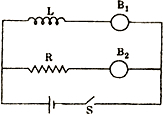Write the mathematical relation for the resistivity of a material in terms of relaxation time, number density and mass and charge of charge carriers in it. Explain, using this relation, why the resistivity of a metal increases and that of a semi-conductor decreases with rise in temperature. Name the gate obtained from the combination of gates shown in the figure. Draw its logic symbol. Write the truth table of the combination.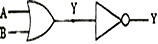Force of attraction between two point charges placed at a distance ‘d’ apart in a medium is ‘F’. What should be the distance in the same medium so that the force of attraction between them becomes 9F ? Two bulbs whose resistances are in the ratio of 1 : 2, are connected in parallel to a source of constant voltage. What will be the ratio of power dissipation in these? Write two properties of a material used as a suspension wire in a moving coil galvanometer. A plane electromagnetic wave of frequency 25 MHz travels in free space along the x-direction. At a particular point in space and time the electric vector is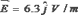. Calculate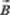at this point. Define the term ‘activity’ of a radionuclide. Write its SI unit. At what angle of incidence should a light beam strike a glass slab of refractive - index √3 , such that the reflected and the refracted rays are perpendicular to each other ? A carrier wave of peak voltage 12 V is used to transmit a message signal. What should be the peak voltage of the modulating signal in order to have a modulation index of 75 %? Which mode of propagation is used by short wave broadcast services having frequency range from a few MHz up to 30 MHz? Derive an expression for the energy stored in a charged parallel plate capacitor with air as the medium between its plates. A bar magnet of magnetic moment 1.5 J/T lies aligned with the direction of a uniform magnetic field of 0.22 T. What is the amount of work required by an external torque to turn the magnet so as to align its magnetic moment,normal to the field direction, & Opposite to the field direction?

Due to paucity of time it would not be possible for us to solve all your queries. We are providing solution to one of your queries. Try solving the rest of the questions yourself and if you face any difficulty then do get back to us.

Two bulbs whose resistance are in the ratio of 1 : 2, are connected in parallel to a source of constant voltage. What will be the ratio of power dissipation in these?

voltage across them is the same.
their respective resistance are : R & 2R
so Power for R = V2/R   , Power for 2R = V2/2R
​ratio 2:1

• -17

u have to take up ur given sums

• -7
What are you looking for?